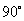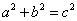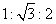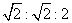How to Learn in 24 Hours?The Rapid Learning Movie

 Need Help? M-F: 9am-5pm(PST): Toll-Free: (877) RAPID-10 US Direct: (714) 692-2900 Int'l: 001-714-692-2900 24/7 Online Technical Support: The Rapid Support Center Secure Online Order:Need Proof? Testimonials by Our Users

 Rapid Learning Courses: MCAT in 24 Hours (2015-16) USMLE in 24 Hours (Boards) Chemistry in 24 Hours Biology in 24 Hours Physics in 24 Hours Mathematics in 24 Hours Psychology in 24 Hours SAT in 24 Hours ACT in 24 Hours AP in 24 Hours CLEP in 24 Hours DAT in 24 Hours (Dental) OAT in 24 Hours (Optometry) PCAT in 24 Hours (Pharmacy) Nursing Entrance Exams Certification in 24 Hours eBook - Survival Kits Audiobooks (MP3)

 Tell-A-Friend: Have friends taking science and math courses too? Tell them about our rapid learning system.

 FAIL (the browser should render some flash content, not this).Home »  Mathematics »  Trigonometry

Geometry Review

 Topic Review on "Title": Angles: An angle is either the figure formed by two rays starting from a common point or the measure of such a figure. Complimentary angles are two angles whose sum is. Supplementary angles are two angles whose sum is. Congruent angles are angles with the same angle measurement. Vertical angles are angles at an intersection of two lines that are not adjacent to each other. Interior angles are two angles that are in the interior of a polygon. Exterior angles are two angles formed by the side of a polygon and an adjacent extends side or two angles on the outside of two parallel lines. Pythagorean Theorem: The Pythagorean Theorem is the sum of the squares for the legs of a right triangle equals the square of the hypotenuse, or. 30-60-90 Right Triangles: The sides of 30-60-90 right triangles are in the ratios of. 45-45-90 Right Triangles: The sides of 45-45-90 right triangles are in the ratios of. Regular polygon: A regular polygon is a geometric figure with three or more sides in which all interior angles are congruent. Area: Area is the size of a planar region or surface of a solid. Volume: Volume is the amount of space taken up by or enclosed by an object.

Rapid Study Kit for "Title":
 Flash Movie Flash Game Flash Card Core Concept Tutorial Problem Solving Drill Review Cheat Sheet"Title" Tutorial Summary : A review of geometric principles is covered in this tutorial. Most geometric relations can be fully understood with the use of the geometric figures and their properties. The formulation and development of area and volume concepts of geometric figures such as triangles and circles is covered in this tutorial. Similarity principles are introduced with the help of special triangles such as the 45-45-90 triangles.

 Tutorial Features: Specific Tutorial Features: • Step by step mathematical examples showing all details: Pythagorean theorem, 30-60-90 right triangles, 45-45-90 triangles, sum of interior angles, area of complex shapes Series Features: • Concept map showing inter-connections of new concepts in this tutorial and those previously introduced. • Definition slides introduce terms as they are needed. • Visual representation of concepts • Animated examples—worked out step by step • A concise summary is given at the conclusion of the tutorial.

 "Title" Topic List: Angles Angles and their definition Relationship of angles and their use Vertical angles Interior and exterior angles Geometric Shapes and Figures Right triangles Pythagorean Theorem 30-60-90 right triangles 45-45-60 right triangles 45-45-90 right triangles Regular polygons Area and Volume Definition of area and volume Area Formulas Volume Formulas

See all 24 lessons in Trigonometry, including concept tutorials, problem drills and cheat sheets:
Teach Yourself Trigonometry Visually in 24 Hours